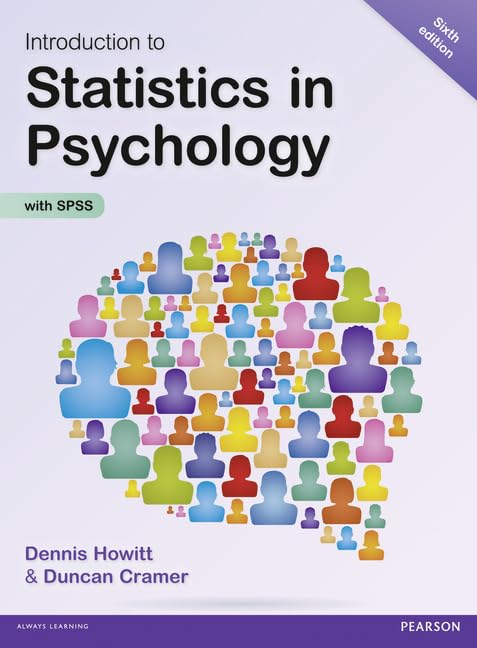Free Shipping in the US# Introduction to Statistics in Psychology by Dennis Howitt

Used Price
\$64.99
Free Shipping in the US

Introduction to Statistics in Psychology, 6th edition is a comprehensive, modern student guide to understanding and using statistics in psychological research. This edition has been significantly revised to incorporate the essential SPSS steps required for carrying out statistical analysis.

Out of stock

## Introduction to Statistics in Psychology Summary

### Introduction to Statistics in Psychology by Dennis Howitt

Introduction to Statistics in Psychology is a comprehensive, modern guide to understanding and using statistics in psychological research. This edition has been significantly revised to incorporate the essential SPSS steps you need for carrying out statistical analysis.

## Customer Reviews - Introduction to Statistics in Psychology

### Why buy from World of BooksOur excellent value books literally don't cost the earthFree delivery in the USEvery used book bought is one saved from landfill

1. Why statistics?

Part 1: Descriptive statistics

2. Some basics: Variability and measurement

3. Describing variables: Tables and diagrams

4. Describing variables numerically: Averages, variation and spread

5. Shapes of Distributions of Scores

6. Standard deviation and z-scores: The standard unit of measurement in statistics

7. Relationships between two or more variables: Diagrams and tables

8. Correlation coefficients: Pearson correlation and Spearman's rho

9. Regression: Prediction with precision

Part 2: Significance testing

10. Samples and populations: Generalising and inferring

11. Statistical significance for the correlation coefficient: A practical introduction to statistical inference

12. Standard error: The standard deviation of the means of samples

13. The t-test: Comparing two samples of correlated/related scores

14. The t-test: Comparing two samples of unrelated/uncorrelated scores

15. Chi-square: Differences between samples of frequency data

16. Probability

17. Reporting significance levels succinctly

18. One-tailed versus two-tailed significance testing

19. Ranking tests: Nonparametric statistics

Part 3: Introduction to analysis of variance

20. The variance ratio test: The F-ratio to compare two variances

21. Analysis of variance (ANOVA): Introduction to the one-way unrelated or uncorrelated ANOVA

22. Analysis of variance for correlated scores or repeated measures

23. Two-way analysis of variance for unrelated/uncorrelated scores: Two studies for the price of one?

24. Multiple comparisons in ANOVA: Just where do the differences lie?

25. Mixed-design ANOVA: Related and unrelated variables together

26. Analysis of covariance (ANCOVA): Controlling for additional variables

27. Multivariate Analysis of Variance (MANOVA)

28. Discriminant (Function) analysis especially in MANOVA

29. Statistics and the analysis of experiments

Part 4: More advanced correlational statistics

30. Partial correlation: Spurious correlation, third or confounding variables, suppressor variables

31. Factor analysis: Simplifying complex data

32. Multiple regression and multiple correlation

33. Path analysis

34. The analysis of a questionnaire/survey project

35. The size of effects in statistical analysis: Do my findings matter?

36. Meta-analysis: Combining and exploring statistical findings from previous research

37. Reliability in scales and measurement: Consistency and agreement

38. Confidence intervals

39. The influence of moderator variables on relationships between two variables

40. Statistical power analysis: getting the sample size right

Part 6: Advanced qualitative or nominal techniques

41. Log-Linear Methods: The analysis of complex contingency tables

42. Multinomial logistic regression: Distinguishing between several different categories or groups

43. Binomial Logistic Regression

Appendices

Appendix A: Testing for excessively skewed distributions

Appendix B1: Large sample formulae for the nonparametric tests

Appendix B2: Nonparametric tests for three or more groups

Appendix C: Extended table of significance for the Pearson correlation coefficient

Appendix D: Table of significance for the Spearman correlation coefficient

Appendix E: Extended table of significance for the t-test

Appendix F: Table of significance for Chi-square

Appendix G: Extended table of significance for the sign test

Appendix H: Table of significance for the Wilcoxon Matched Pairs Test

Appendix I: Table of significance for the Mann-Whitney U-test

Appendix J: Table of significance values for the F-distribution

Appendix K: Table of significant values oft when making multiple t-tests

Glossary

References

Index

GOR007067664
Introduction to Statistics in Psychology by Dennis Howitt
Dennis Howitt
Used - Very Good
Paperback
Pearson Education Limited
2013-12-17
744
1292000740
9781292000749
N/A
Book picture is for illustrative purposes only, actual binding, cover or edition may vary.
This is a used book - there is no escaping the fact it has been read by someone else and it will show signs of wear and previous use. Overall we expect it to be in very good condition, but if you are not entirely satisfied please get in touch with us.